# Automatic Expansion

When you insert rows (or columns) at the end of a formula range the formulas (by default) will be expanded automatically.
Formulas will only expand automatically when you insert rows (or columns) at the end of a formula range when the following option is selected.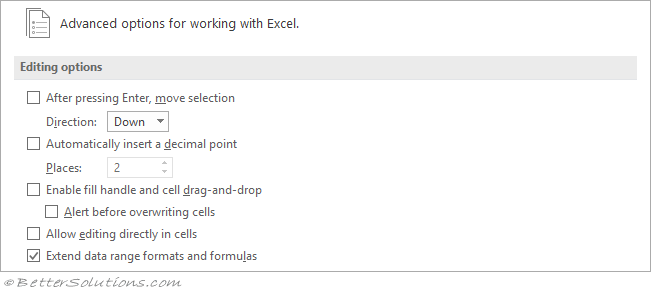### Extend Data Range Formats

If an AutoFormat has been applied to the list then the format is not automatically extended
Dates are not automatically formatted
Any data pasted from the clipboard will not have automatic formats applied to it.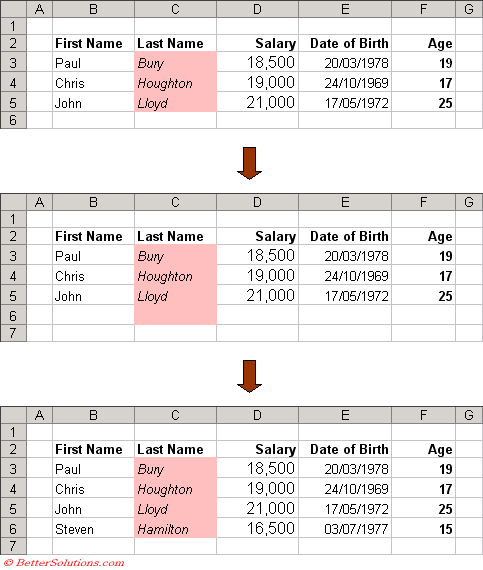### Extend Formulas

Formulas will not be automatically expanded when you insert rows (or columns) at the start of a formula range.
Formulas will not be automatically expanded (or copied) when you paste data from the clipboard.

### Extend Formulas - Copying - Typing at the End

Excel will automatically copy formulas when you add new data to a table if the same formula appears in four or more consecutive rows or columns.
Typing May below the table and inserting numerical data into cells "C7", "D7" and "E7" will cause the formula in column "F" to be automatically copied to cell "F7".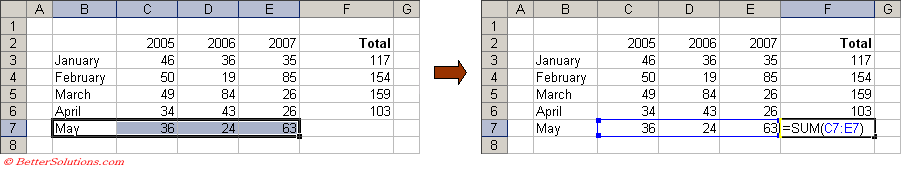### Extend Formulas - Inserting in the Middle

Formulas are always automatically expanded when you insert new rows and columns inside existing formulas.
Inserting a row above row 5 (i.e. in the middle) will cause the SUM() formula to automatically expand.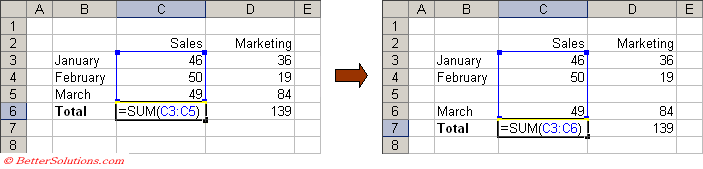The same is true when you have formulas that refer to columns and you insert new columns inside existing formulas.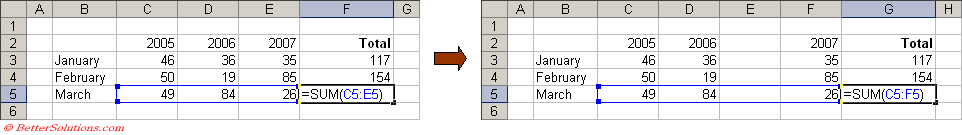In these situations the formulas will always expand (automatically) and there is no option to turn this off.

### Extend Formulas - Inserting - At The End

Excel will automatically expand formulas at the end of a formula range if they refer to three or more consecutive rows or columns.
Inserting a row underneath March and entering a number into cell "C6" will cause the SUM() formula in cell "C7" to automatically expand.
The SUM() formula will only expand automatically if you enter numerical data into cell "C6".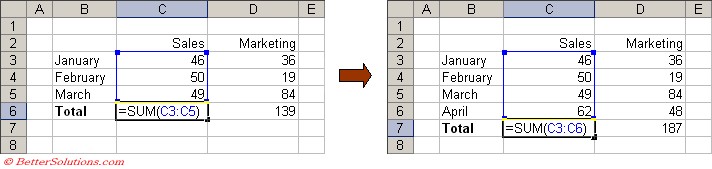Formulas will not be expanded automatically if you insert rows (or columns) at the start of a formula range.